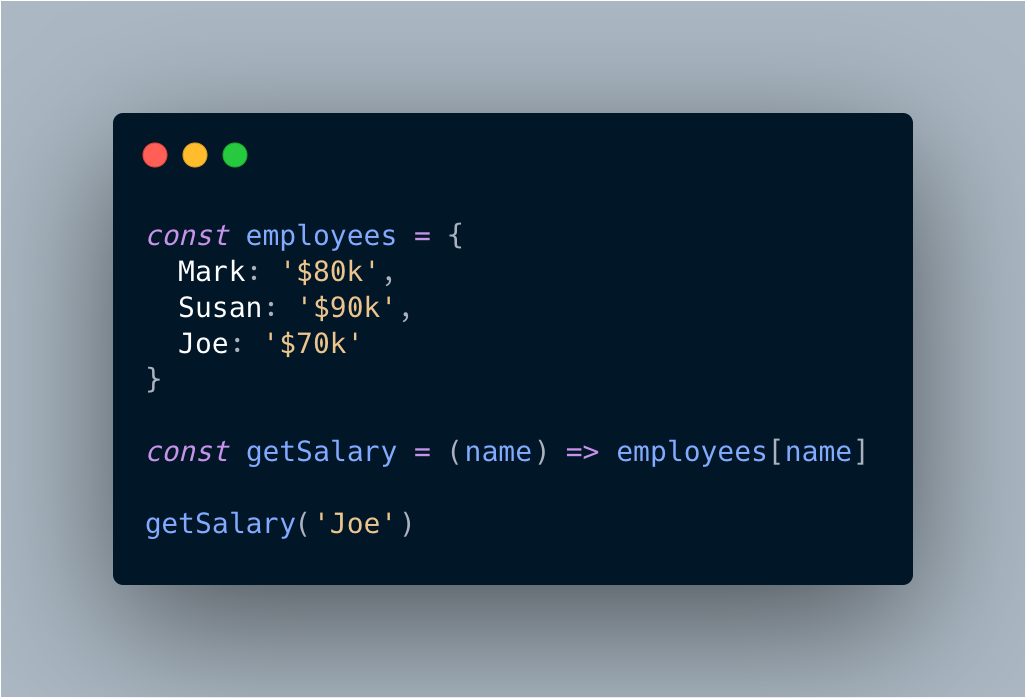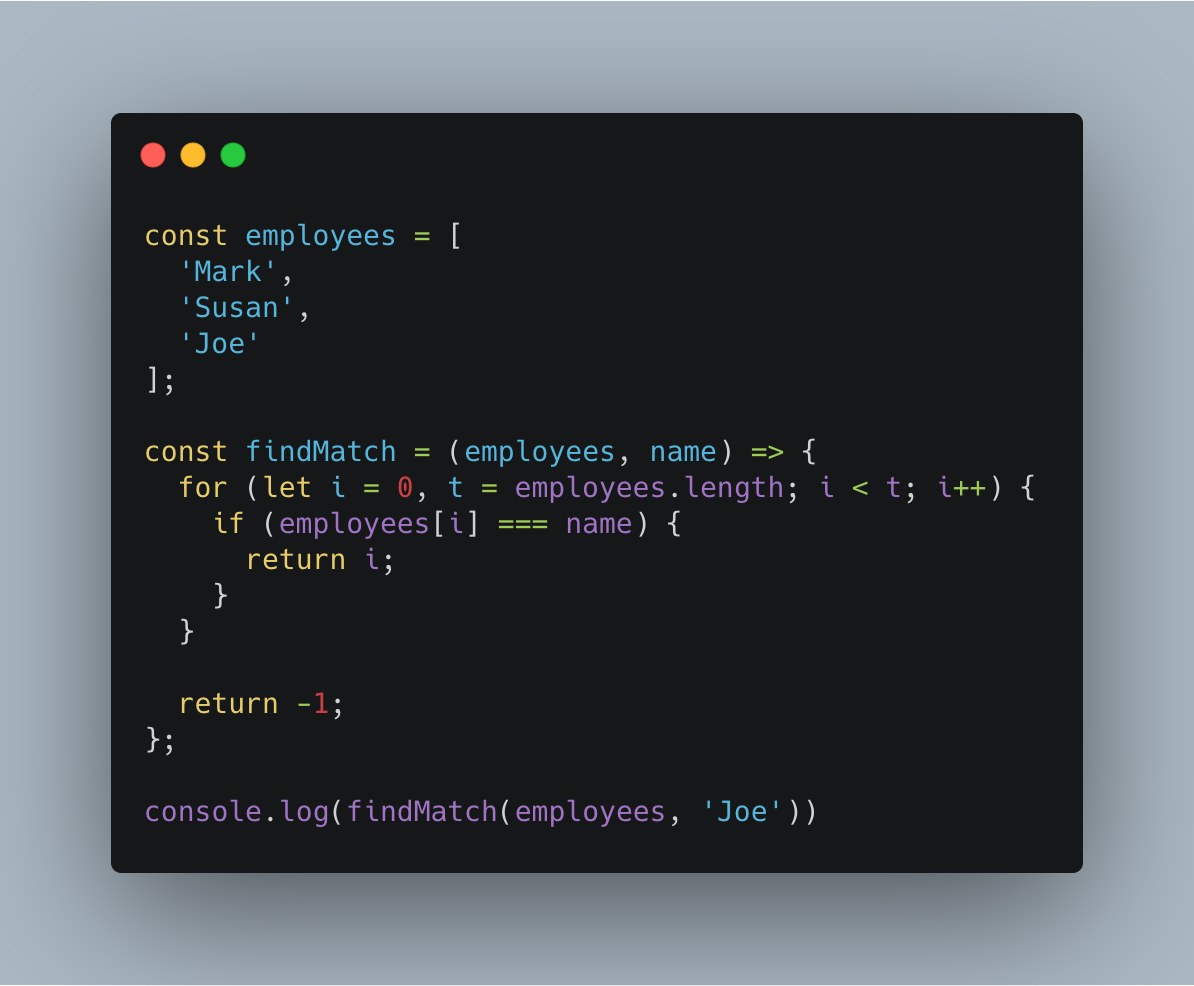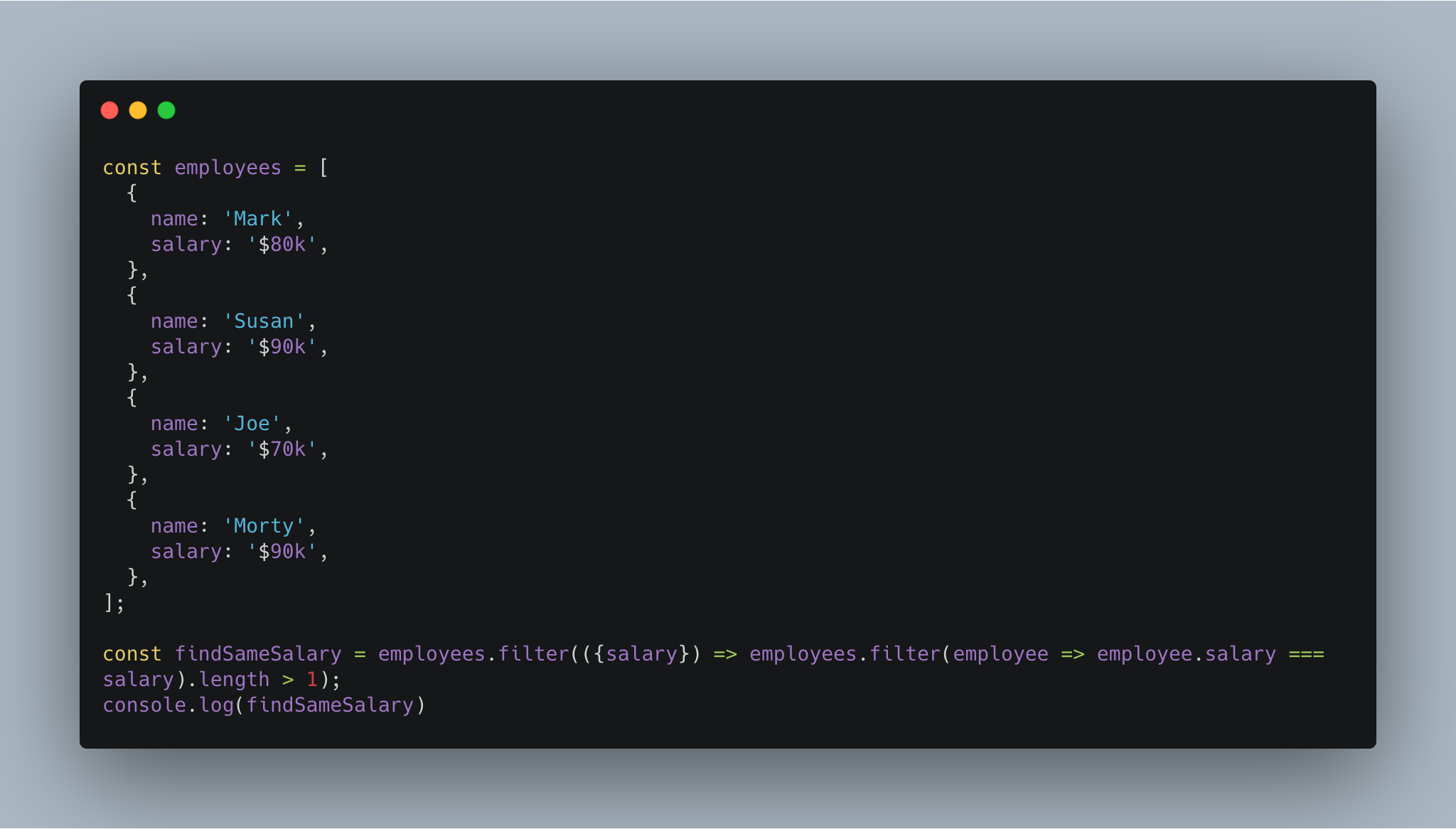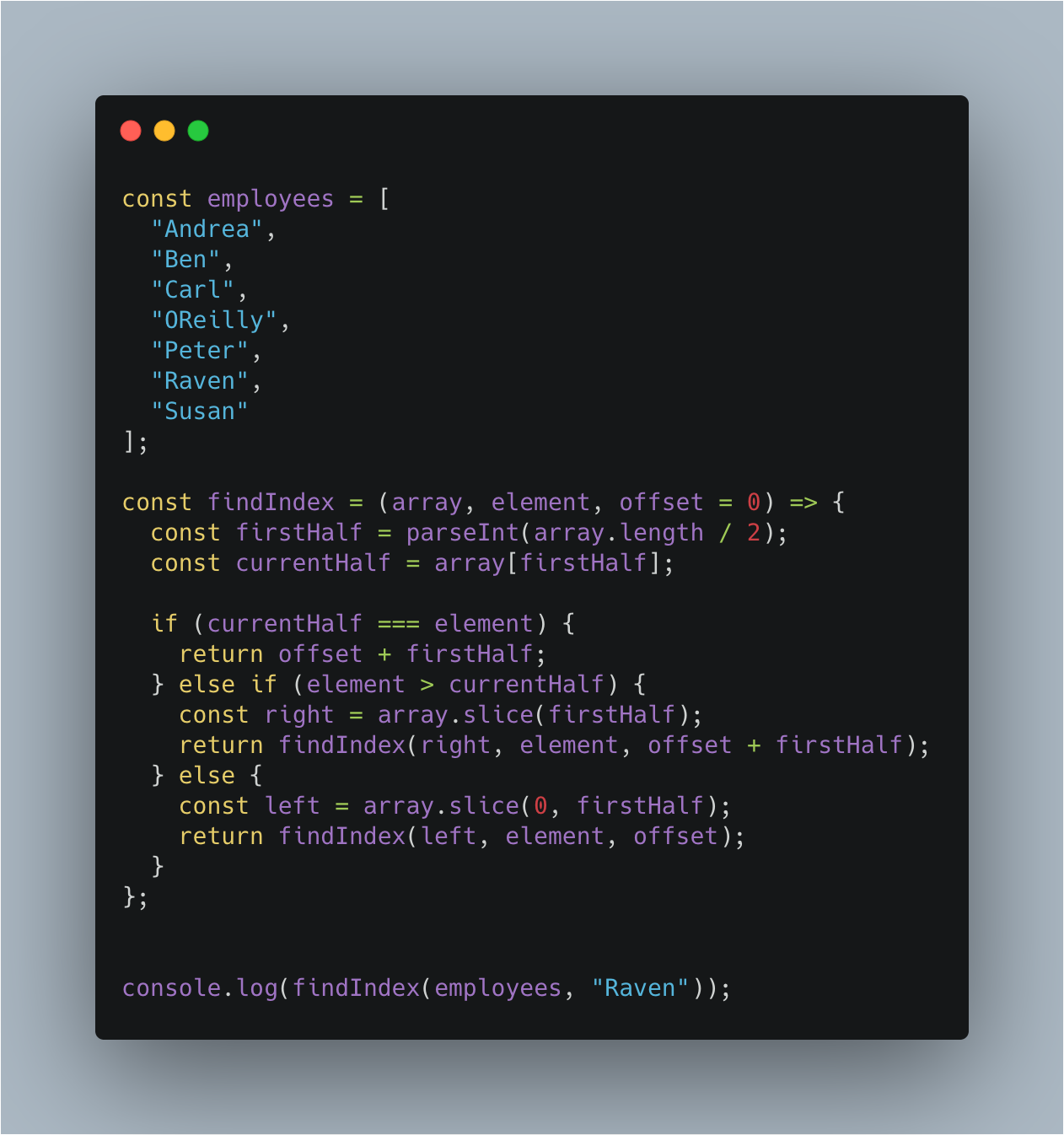# Understanding Time complexity - Big O Notations

Lately, I have gotten an interest in algorithms, the first one I chose to understand deeply is how sorting algorithms work and their time complexity. However, this post is not to explain sorting algorithms, instead, we will take a step back and understand time complexity [Big O Notation] in the simplest way possible.

### Before we go any further, let's understand what is an Algorithm:

An Algorithm is a step by step instruction, telling a program to execute in a certain way to solve a particular problem. And it's pretty obvious, that when we run a program in any language, it will have its own execution time depending on various factors such as input, operations being performed, etc.

#### Now the next question would "What is Time complexity"?

Time complexity is the execution time it takes for your algorithm to solve a problem. Simple, right?.

To explain it a little further, the time complexity is driven by two things i.e. execution time & the space required by the program.

#### Why do we need to measure the time complexity?

As programmers, when we write a program, it's kind of important to understand the different operations we are performing which can be checked by measuring the complexity. Normally, the execution time is taken for granted, we don’t care about mi-nute calculations which are happening behind the scene. So, overall, time complexity helps us to improve the efficiency of the code we’ve written.

#### How do we measure time complexity?

The answer is Big O Notation when the letter O means `Order of the program`.

Big O Notation (a mathematical expression) helps to measure the time complexity by classifying how your program behaves with varying input and taking in different operations.

Let’s understand the common types of notations, we will use Javascript here to explain with examples, although the idea is similar for different languages.

### Types of Big O Notations:

• Constant-Time Algorithm - O (1) - Order 1:

This is the fastest time complexity since the time it takes to execute a program is always the same. It does not matter that what’s the size of the input, the execution and the space required to run this will be the same.

For example: Take a case of simple array lookup or getting the last item of the array.The above example will always go through the array once and finds the salary of an employee called `Joe`. That means, only one iteration is involved i.e `O(1)`.

• Linear-Time Algorithm - O(n) - Order N:

Linear Time complexity completely depends on the input size i.e directly proportional. One of the examples could be just printing the elements from an array OR finding a particular match in an array.

While calculating, we should always think of both the “best” & “worst” scenario.

For example: If we were to match a specific element in an array, then it can be the very first one or the last one, so in that case, we can assume its O(n). Let’s take an example here:• Quadratic-Time complexity - O(n2) - Order N Squared:

As the name suggests, the time to execute a program is proportional to the square of the input size. That means, in our program, when we are trying to perform two operations which are kind of a combination of Linear and Constant time complexity, then those are called Quadratic-time complexity.

This type of complexity is commonly used with sorting algorithms.

Let’s try to understand by taking an example:In this example, it is clear that we first have a single filter loop at the top iterating over an array once, then we have a nested loop that is looking for similar salaries of the employees by iterating through the array again.

• Logarithmic-Time Algorithm - O(log n) - Order log N:

This is considered the most efficient way to deal with a large number of data in a set. The idea behind this approach is to divide the data into chunks and then perform operations. A logarithm is basically a quantity that represents the base power, that means if the data grows logarithmically then it's literally being divided.

For example, if we want to find a salary of a few employees from 50 records, then that means we usually have to go through each record and look for it. Assuming if we use `log base 2`, we would be able to find it in `log2(50) = ~6` iterations. That is a lot of power!

Its commonly used with different sorting algorithms such as QuickSort, Merge-sort which is usually used to find an element or sort a list. Or binary Search is a good example.I think we've covered most commonly used notations. If you would like to read more about it, I'd refer few good links to go through: